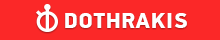GMAT Question of the Day - Daily to your Mailbox; hard ones only

 It is currently 26 Jun 2019, 11:26GMAT Club Daily Prep

Thank you for using the timer - this advanced tool can estimate your performance and suggest more practice questions. We have subscribed you to Daily Prep Questions via email.

Customized
for You

we will pick new questions that match your level based on your Timer History

Track

every week, we’ll send you an estimated GMAT score based on your performance

Practice
Pays

we will pick new questions that match your level based on your Timer History

Not interested in getting valuable practice questions and articles delivered to your email? No problem, unsubscribe here.If cement, gravel, and sand are to be mixed in the ratio 3:5:7 respect

Author Message
TAGS:

Hide Tags

Math ExpertV
Joined: 02 Sep 2009
Posts: 55803
If cement, gravel, and sand are to be mixed in the ratio 3:5:7 respect  [#permalink]

Show Tags00:00

Difficulty:45% (medium)

Question Stats:57% (01:22) correct43% (01:39) wrongbased on 93 sessions

HideShow timer StatisticsIf cement, gravel, and sand are to be mixed in the ratio 3:5:7 respectively, and 5 tons of cement are available, howmany tons of the mixture can be made? (Assume there is enough gravel and sand available to use all the cement.)

A. 15
B. 20
C. 25
D. 30
E. 75

_________________
Senior ManagerP
Joined: 17 Oct 2016
Posts: 259
Location: India
Concentration: General Management, Healthcare
GMAT 1: 640 Q40 V38GMAT 2: 680 Q48 V35GPA: 3.05
WE: Pharmaceuticals (Health Care)
If cement, gravel, and sand are to be mixed in the ratio 3:5:7 respect  [#permalink]

Show Tags

Bunuel wrote:
If cement, gravel, and sand are to be mixed in the ratio 3:5:7 respectively, and 5 tons of cement are available, howmany tons of the mixture can be made? (Assume there is enough gravel and sand available to use all the cement.)

A. 15
B. 20
C. 25
D. 30
E. 75

the mixture is in ratio 3:5:7 , so with 5 tons of cement, ratio becomes 5:x:y (where X and Y are the total quantities of gravel and sand)

3/5 = 5/x; X = 25/3

and

3/7 = 5/y; Y = 35/3

Total mixture = 5 + 25/3 + 35/3
= 5 + 60/3
= 5 + 20
= 25

_________________
_____________________
Chasing the dragon
CEOP
Joined: 18 Aug 2017
Posts: 3947
Location: India
Concentration: Sustainability, Marketing
GPA: 4
WE: Marketing (Energy and Utilities)
Re: If cement, gravel, and sand are to be mixed in the ratio 3:5:7 respect  [#permalink]

Show Tags

1
Bunuel wrote:
If cement, gravel, and sand are to be mixed in the ratio 3:5:7 respectively, and 5 tons of cement are available, howmany tons of the mixture can be made? (Assume there is enough gravel and sand available to use all the cement.)

A. 15
B. 20
C. 25
D. 30
E. 75

given 3:5:7
and 5:x:y ratio of cement: gravel:sand

we can say 3/15= 5/5+x+y
x+y=20

similarly for gravel
5/15= x/25 or say x= 25/3
and sand
7/15=y/25 or say y= 35/3
total ; 35/3+25/3+5 = 75/3= 25 IMO C
_________________
If you liked my solution then please give Kudos. Kudos encourage active discussions.
InternB
Joined: 16 Jul 2018
Posts: 38
Re: If cement, gravel, and sand are to be mixed in the ratio 3:5:7 respect  [#permalink]

Show Tags

Quote:
If cement, gravel, and sand are to be mixed in the ratio 3:5:7 respectively, and 5 tons of cement are available, howmany tons of the mixture can be made? (Assume there is enough gravel and sand available to use all the cement.)

A. 15
B. 20
C. 25
D. 30
E. 75

So... 3x+5x+7x=15x,
3x=5
x=5/3
15*5/3=25
VPP
Joined: 07 Dec 2014
Posts: 1198
Re: If cement, gravel, and sand are to be mixed in the ratio 3:5:7 respect  [#permalink]

Show Tags

Bunuel wrote:
If cement, gravel, and sand are to be mixed in the ratio 3:5:7 respectively, and 5 tons of cement are available, howmany tons of the mixture can be made? (Assume there is enough gravel and sand available to use all the cement.)

A. 15
B. 20
C. 25
D. 30
E. 75

5/3*(3+5+7)=25
C
GMAT TutorB
Joined: 07 Nov 2016
Posts: 56
GMAT 1: 760 Q51 V42Re: If cement, gravel, and sand are to be mixed in the ratio 3:5:7 respect  [#permalink]

Show Tags

1
Bunuel wrote:
If cement, gravel, and sand are to be mixed in the ratio 3:5:7 respectively, and 5 tons of cement are available, howmany tons of the mixture can be made? (Assume there is enough gravel and sand available to use all the cement.)

A. 15
B. 20
C. 25
D. 30
E. 75

In ratio terms cement = 3, and total mixture = 3 + 5 + 7 = 15

i.e the total mixture is 5 times the quantity of cement

If cement = 5 tons, total mixture will be 5 *5 = 25 tons

Choice C
_________________
Math ExpertV
Joined: 02 Aug 2009
Posts: 7765
Re: If cement, gravel, and sand are to be mixed in the ratio 3:5:7 respect  [#permalink]

Show Tags

1
1
Bunuel wrote:
If cement, gravel, and sand are to be mixed in the ratio 3:5:7 respectively, and 5 tons of cement are available, howmany tons of the mixture can be made? (Assume there is enough gravel and sand available to use all the cement.)

A. 15
B. 20
C. 25
D. 30
E. 75

We do not have to get into too much of calculations

If we take common ratio to be X..
C:G:S becomes 3x:5x:7x...
Now 3x=5...
Now, don't complicate by taking value of x and substituting in total
Just add the total value => 3x+5x+7x=15x=5*(3x)=5*(5)=25

C
_________________Re: If cement, gravel, and sand are to be mixed in the ratio 3:5:7 respect   [#permalink] 13 Jan 2019, 21:26
Display posts from previous: Sort by

If cement, gravel, and sand are to be mixed in the ratio 3:5:7 respect